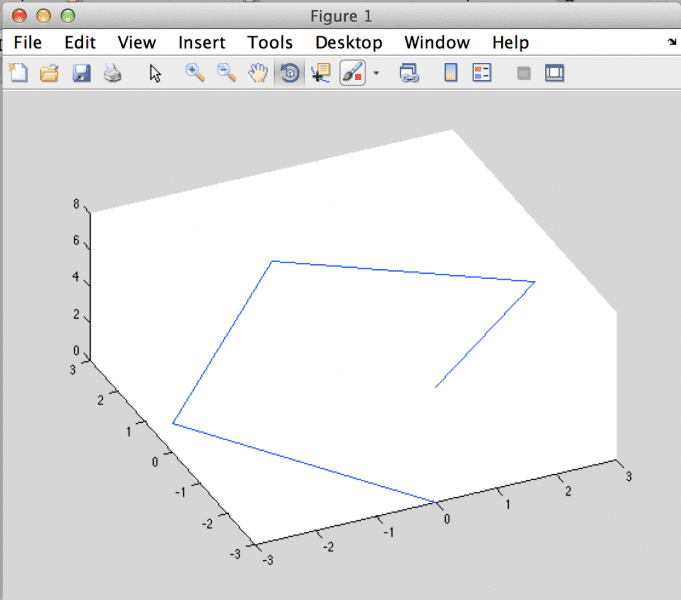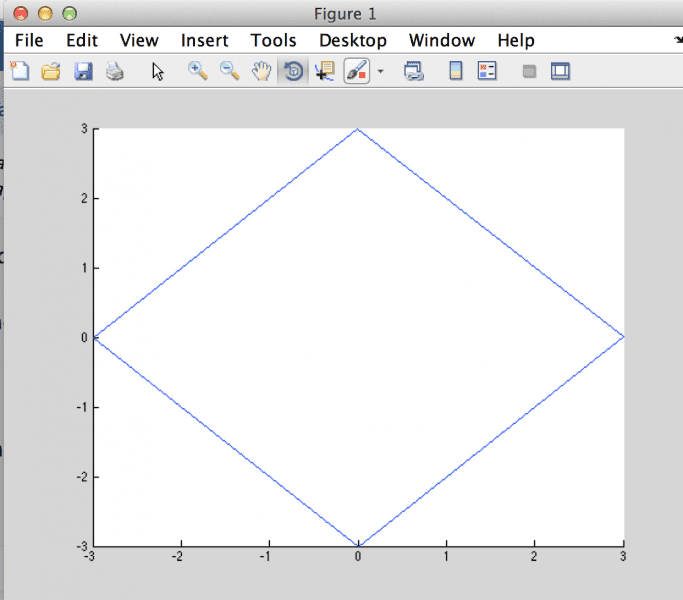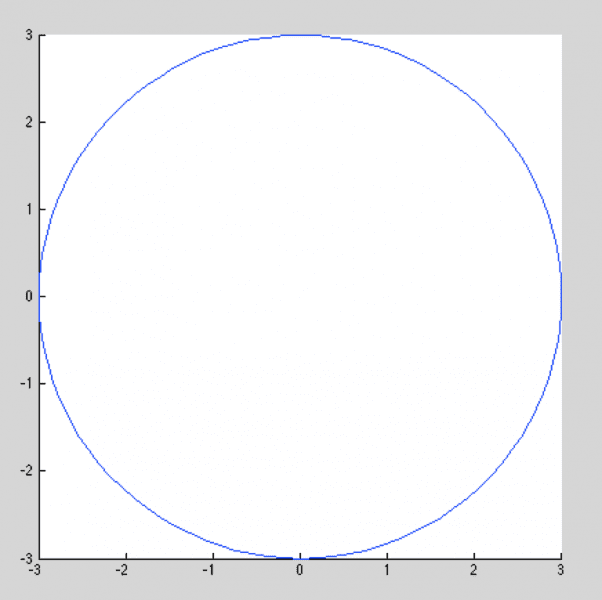# Parametric equation of a particle in a circular trajectory

leo255

## Homework Statement

[/B]
You are given a particle, with the trajectory of a circle, with radius of 3 meters. The particle moves clock-wise, and when t=0, the x, y coordinates are (0, -3). Find parametric equations for the coordinates (x, y) in terms of the parametric t (time).

## The Attempt at a Solution

My attempt at a solution was to plot the circle with the given points. I was able to determine that x = -3sin, and that y = -3cos. I know that period equals 2 pi / B, but I'm not sure what to do here for the last part of the trig equations.

Edit: Just remembered that the period should be 4. So, B = pi/2.

Would the correct parametric equations be

x = -3sin(pi/2) t
y = -3cos(pi/2) t

Last edited:

Homework Helper
I got the same result.

Mentor
Edit: Just remembered that the period should be 4
Was this something that you forgot to put in the original problem statement? Otherwise, there is nothing in the problem statement that says anything about the period.

Homework Helper
You have a circle ##x^2 + y^2 = 9## of radius ##3## which passes through the point ##(0, -3)## at ##t = 0##.

The parametrization you have given is on the right track.

Replace the ##\frac{\pi}{2}## you have there with the ##t## you seem to have left out of the argument.

leo255
Thanks all for the help. Still slightly confused about why we replace pi/2 with t. Isn't the period equal to 4, since both trig functions complete their shapes in t = 4?

Homework Helper
Thanks all for the help. Still slightly confused about why we replace pi/2 with t. Isn't the period equal to 4, since both trig functions complete their shapes in t = 4?

You're probably better off plotting in radians rather than plain numbers.

Try finding values of ##x(t)## and ##y(t)## for the points ##t = 0, \frac{\pi}{2}, \pi, \frac{3 \pi}{2}, 2 \pi##.

Do those points match up to your circle over in the ##(x, y)## plane?

What can you conclude?

Edit: Out of boredom I created this useful MATLAB session to assist the visualization. Here is a parametric plot of ##x(t)##, ##y(t)## where I've chosen some useful points to plot:Simply rotating the picture so that the ##(x, y)## plane is in your front view, you can see the points in the plane:In fact, allowing the interval ##[0, 2 \pi]## to become dense, you obtain something like this:Last edited:
•leo255
leo255
Thank you! That makes a lot more sense. The visuals help a lot.